# Team:ITB Indonesia/Modeling/Difussion

## Difussion

First thing to do is calculate how much aflatoxin would enter the cell every time. Aflatoxin diffused into cell through simple diffusion mechanism, it means that aflatoxin difusion is drived by concentration gradient.

To simplify our model, we assume that aflatoxin homogenely diffused into cell

0. General equation of diffusion through membrane
Diffusion is frequently modelled if the system needs to transport some molecule through cell membrane. Mathematical model for diffusional phenomena through membrane is :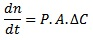Where n represents the number of molecule involved in diffusion (So, dn/dt can be stated as “molecule flux through cell membrane”). Parameters of the equation :

 Variable Definition Value Source P Permeability aflatoxin-membrane E. coli 1,01 x 10-4 cm/s Calculated (See Sect 1) A E. coli membrane cell area Changing with time Calculated (see Sect 2) dC Concentration gradient between inner and outer side of the cell - Depends on case

I. Permeability aflatoxin-membrane
Permeability value between solute and solvent is very specific for each case, and finding an analogous case to our system is really difficult. We try to tinker some diffusional equation to find permeability between aflatoxin and membrane with really few data.
Generally, permeability can be described through this equation :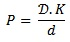Variable Definition Value Source D Diffusivity constant of aflatoxin-membrane E. coli 2,05 x 10-9 cm2/s Calculated (see below) K Partition constant of aflatoxin-membrane E. coli 0,64  d E. coli membrane thickness 13 nm 

To determine the value of diffusivity constant, we use Stoke-Einstein equation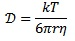where the radii value of solute (r) can be determined with the help of molecular weight data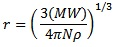So, to simplify our equation, we try to find the correlation between diffusivity constant and solute molecular weight. It can be done through dividing two sets of case (diffusion of protein with well-known molecular weight and diffusion of aflatoxin) and the result is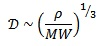Variable Definition Value Source ρ Aflatoxin density 1,64 g/cm3  MW Aflatoxin molecular weight 312,3 

How we did it in Simbiology?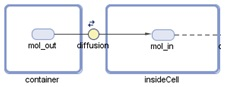We create a reversible reaction with customized reaction rate (‘Unknown’ kinetic law), where k_permin as forward reaction rate and k_permout as backward reaction rate. k_permin and k_permout is stated by repeatedAssignment rule :
k_permin = P*Acell*((container.mol_out/container) - (insideCell.mol_in/insideCell))
k_permout = P*Acell*(-(container.mol_out/container) + (insideCell.mol_in/insideCell))
Notice that k_permin and k_permout is the same as dn/dt in membrane diffusion general equation

II. Aflatoxin membrane cell area
When we discussed with team, there is still one problem in this model :

“Our biosensor is a live device and can keep replicating even in the middle of analysis process. How it will affect this model?”

Good thinking! To simulate the effect of cell growth to diffusion phenomena, we modify the diffusion equation. Membrane cell area will be increased along with cell number, and it affected by cell growth.

We simplify this problem by assuming cell growth is like growing sphere. When the cell number doubled, it can be stated that the membrane cell area and cell volume doubled too.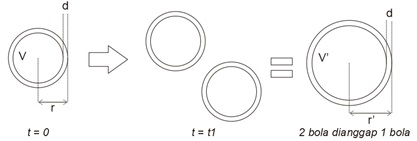Cell membrane area can be evaluated every time by this equation :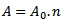where the value of A0 represents membrane cell area of one E. coli cell and n is cell number at certain time. The value of n can be determined with cell growth kinetic :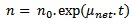So, the equation’s final form to evaluate cell membrane area every time become :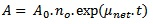With the same principle, we can evaluate cell volume every time :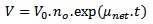To gather the value of A0 dan V0, we use the data that bacteria has area to volume ratio 3:1 . Cell density and wet cell mass of E. coli can be known from literature

 Variable Value Source Cell density 1,105 g/ml  Wet cell mass 10-12 g 

So the value of A0 and V0 is :

 Variable Value A0 2,715 x 10-18 m3 V0 9,05 x 10-19 m3

How we did it in Simbiology?
Uncheck the “Constant Value” in parameter A (cell area) and insideCell (cummulative cell volume). Then we apply this rule (because we use “rate” as rule type, the equation must be written in the form of first derivative or dx/dt) :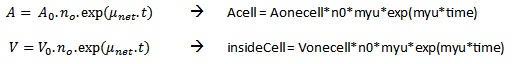Another perspective from our model instructor, Mochamad Apri, said that involving cell growth into diffusion equation will be valid if our system’s analysis time is far more greater than cell growth rate. If our system’s analysis time is below cell growth rate, it will be wise to assume that cell growth is negligible for diffusion phenomena.

III. Average aflatoxin concentration in each of our cell
On previous section, we simulate diffusion phenomena by assuming all cell is gathered into one big cell with volume = n*Vcell and membrane area = n*Acell. How we calculate aflatoxin concentration in each of our cell? The answer is simple, just divide aflatoxin molecule in “big cell” with cell number at recent time. But how can we do this simple division in Simbiology? Simbiology is designed to handle reaction equation, and we need another way to do this simple equation.
After long time searching, the solution is really easy. We just copy the same reaction rate from diffusion phenomena to this “distribution” reaction (actually there is no such reaction in real world, it just helped us find the average aflatoxin concentration) and divide it by cellNum (parameter for cell number).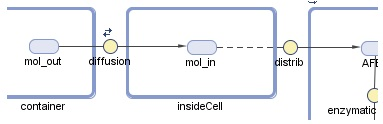diffusion reaction rate                   : k_permin – k_permout
distrib reaction rate        : (k_permin – k_permout)/cellNum

We hope this will help other team who face the same problem with us.